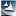# 第一部分：惯性力本质的明确解释科学网—惯性力本质的明确解释 - 陈驰一的博文20160110.png１，惯性系 $O$ ：   $\vec{F}_{_{p}}=m_{p}\vec{a}_{p-O}$

２，非惯性系（即实际参考系） ${O}'$ ：   $\vec{F}_{p}+\vec{f}_{inertial}=\vec{F}_{p}-m_{p}\vec{a}_{_{{o}'-o}}=m_{p}\vec{a}_{_{p-{o}'}}$

$\vec{F}_{_{p}}-\frac{m_{p}}{m_{O}}\vec{F}_{_{O}}=m_{p}\vec{a}_{p-O}$   直接适用于任何无自转的实际参考系.

补充解释

$\vec{F}_{1}-\vec{F}_{2}=m\vec{a}_{1}-m\vec{a}_{2}=m\vec{a}_{1-2}$ ,

$\vec{F}_{1}=m\vec{a}_{1-2}$ 。1，物理上因果一致性的要求

2，数学上定义的要求：位置矢量的定义至少必须给定两个空间点P和Ｏ。

3，实践上：更好符合经典力学实验

4，美学上：体现自然秩序的对称美和宇宙根本法则的万物平等

https://blog.sciencenet.cn/blog-96769-829150.html

## 相关博文

GMT+8, 2021-12-4 04:39